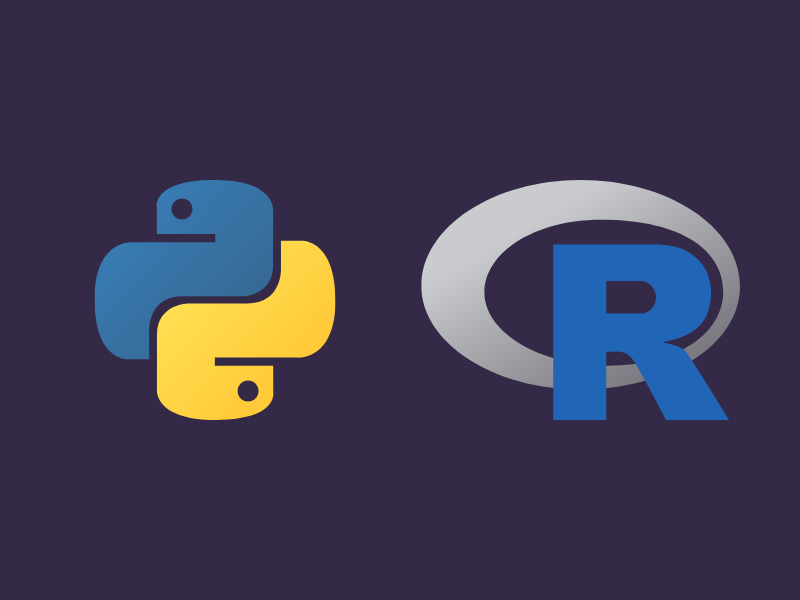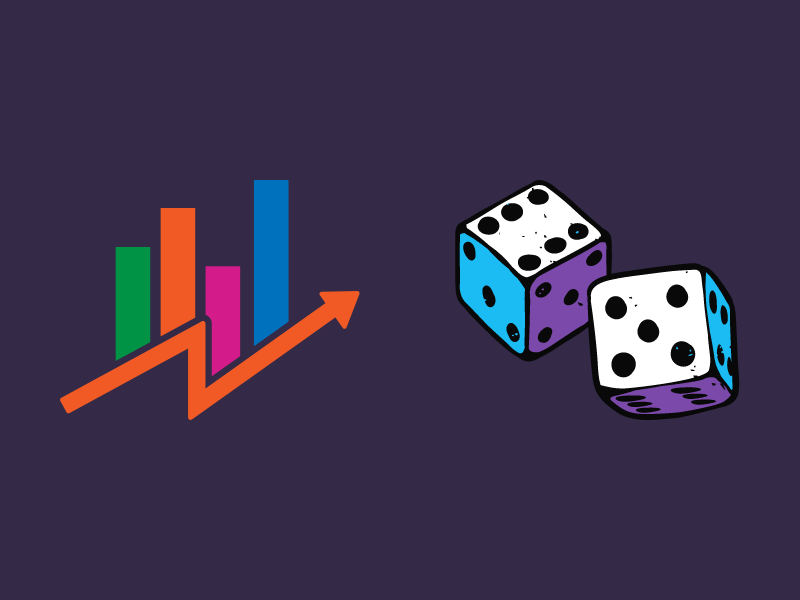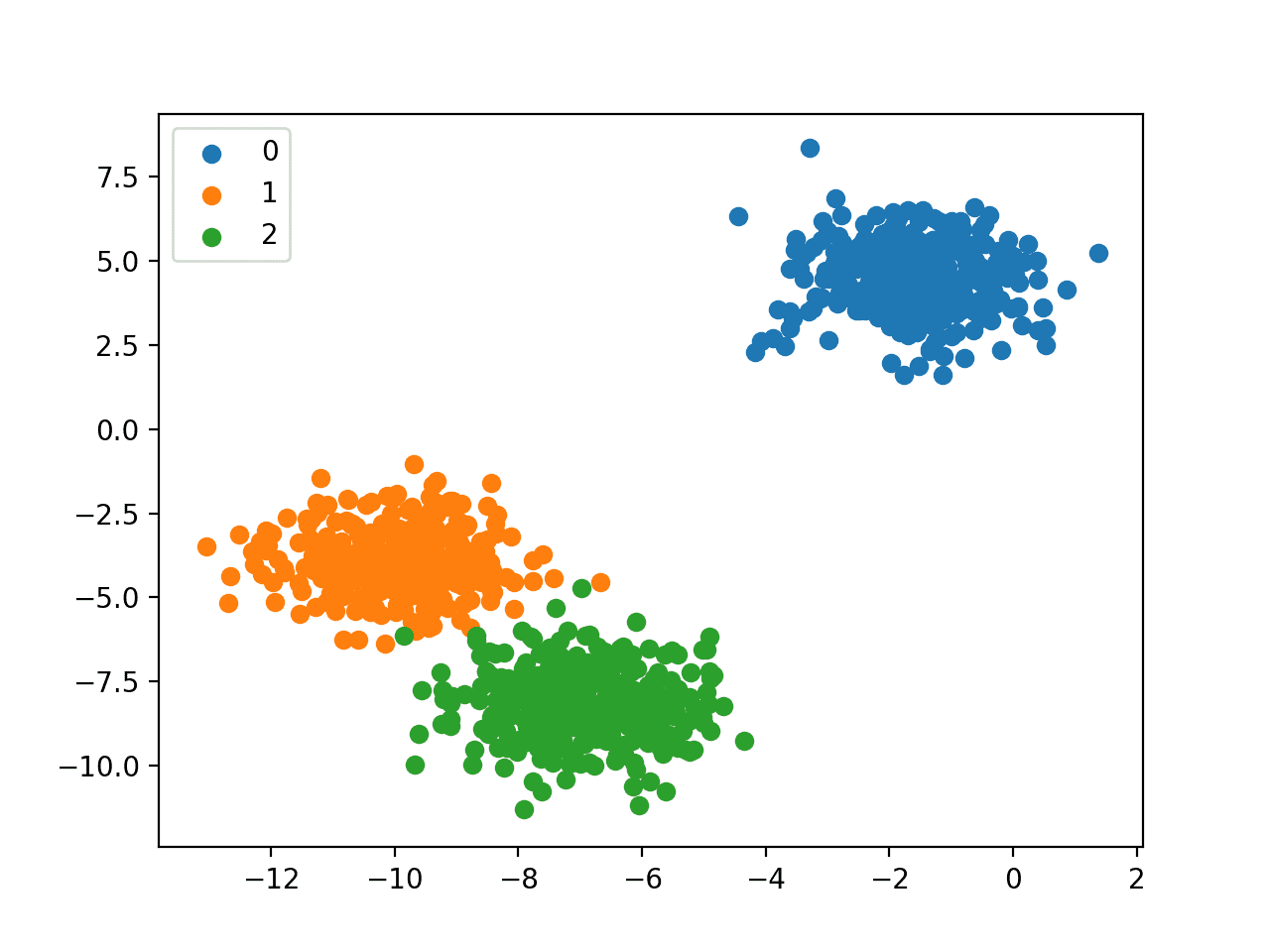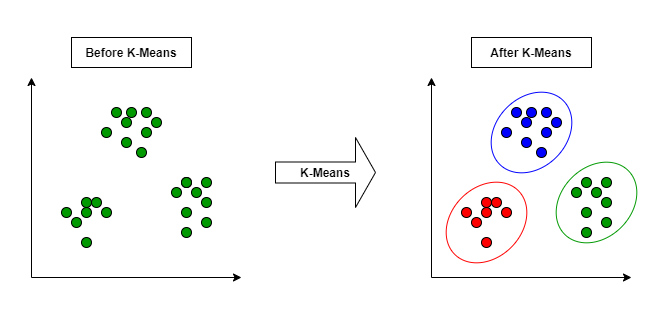×

Search anything:

# Getting started with Data Science

#### data scienceGet this book -> Problems on Array: For Interviews and Competitive Programming

Every day in this world data is generating at the rate of 2.5 quintillion (2,500,000,000,000 millions) bytes per second. The field of Data Science tries to explore the insights we can gather from the data how can we predict the future or how one organization can improve its efficiency by acting on that data. Data Science inspects many techniques through which we gather data, clean it, transform it, gain insights and even predict future outcomes. It is one of the most recommended job of the century.

# Programming Languages:There are 2 major Programming languages that are in use in this industry:

1. Python
2. R

Python is one of the most used language in this field due to availability of its vast libraries which makes the job easier in the end. Learning basics of python is a necessity with an addition of learning both pandas and NumPy library. Pandas will help you in manipulating data in a CSV/Excel file. While NumPy will help you in performing arithmetic operations.

R is used for statistical modelling and data visualization. If your goal is statistical modelling and data visualization then you could learn R. The "ggplot2" library contains strong tools that can help you visualize data more efficiently than the python's "matplotlib" library.

Now the programming part's covered we have now to learn Statistics and Probability. From my part I would recommend to go for python as it is the most widely used language in the industry

# Statistics and Probability:## Probability:

Probability is a much needed concept in this field. It is process of finding the odds of an outcome to occur. There are many important concepts you should learn before you start the Data Science journey.

Some concepts are: Random Variable, Calculating Probability by principle of counting, binomial distribution, continuous random variables, central limit theorem, area under normal distribution and Z-score.

## Sampling:

Sampling is the process of picking out the right set of data. Suppose if we have a dataset of multiple countries who are in poverty and we have to find how many people are in below poverty line in a particular country so we will sample out the data of that particular country i.e. picking out the right set of data.

## Distribution of Data:A distribution represents all possible values of the data on a single graph. It is like a Normal Distribution. A normal distribution has mean, mode and median that all conincides at one peak.

## Hypothesis Testing:

Hypothesis testing is done based on an assumption based on the population(data) we have. It determines whether the given action that we will perform will have outcome as a positive result or null hypothesis. When a hypothesis is proved false it is called as a null hypothesis.

## Variation Testing:

Variations are the errors, distortions or outlier in the data. Important topics to learn are: Range, Variance, Error Deviation, Standard Deviation, Covariance, Correlation, etc.

# Learn Pandas:

Pandas is a python library that is used for data manipulation in a DataFrame. A DataFrame is a tabular data set containing data in the form of rows and columns. It includes functions for reading and writing data, handling missing data, filtering data, merging data, vizualising data, etc. To learn pandas you can visit to this link.

# Learn Machine Learning:

We have covered Data Analytics with the above topics. Now we will see with the help of machine learning how we can predict results from the upcoming data.

In Python we use Scikit-Learn library to perform Machine Learning operations on data. It is one of the most popular library in Python.

First the data is splitted into 80:20 ratio. It works as a hit and miss function. The model is trained on 80% of the data and then the model is tested on the remaining of 20% of the data.

Then the accuracy of the model is calculated. There are multiple methods of doing that based on the type of model you are creating.

You can learn Scikit-Learn using this link

There are two types of Machine Learning Algorithms:

1. ̥Supervised Machine Learning: The prediction is relied upon labeled data.
2. Unsupervised Machine Learning: The prediction is relied upon unlabeled data.

There are three key Machine Learning algorithms in Data Science:

1. ̥Regression
2. Classification
3. Clustering

## Regression:

This method explains the relationship between one independent variable and one dependent variable on a straight line. It comes under the category of supervised Machine Learning algorithms. It also helps in forecasting as we can see that the predicted data should be near to the straight line that has been created.

Applications: Stock Market Prediction and House Price Prediction

## Classification:Classification classifies your discrete data into multiple classes. To take as an example imagine there are four people in a room. Now, I want to identify speech of these four people. To identify it I will classify that data into four different category so that we can identify speech of every person in that room. This process is part of supervised learning.

Applications: Sentiment Analysis, Image Classification, Speech Recognition, etc.

## Clustering:It is a technique of grouping of unlabeled data. It finds certain patterns that are very similar to some data points and then groups them into single cluster. It is a method of unsupervised machine learning. It deals with the unlabeled dataset.

Applications: Market Segmentation, Statistical Analysis, Social Network Analysis, etc.

# Where to practice your Learning?

1. Kaggle: This is one of the best website for Data Science enthusiasts. This website has a large library of datasets. It has its own course tracks where you can learn from Python to Machine Learning. It has its own competions where you can even earn good prizes.
2. OpenGenus IQ: Go through Data Science topics on the site to stay on track with the latest trends.
3. Github: You can contribute to open source projects on Github where you will get the exposure of real world problems that developers are trying to solve.
4. Make your own projects: Start with a simple one but as you learn try to make a project that is original and unique in a way that is solving a very big problem in people's life. See some project ideas for Data Science.